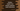# Python program to check two integer arrays contains same elements## Python program to check two integer arrays contains same elements:

In this post, we will learn how to check two integer arrays hold the same elements. Both arrays are of equal size and the order of the elements can be different.

For example, array [1,2,3,4,5] and [5,4,3,2,1] are considered equal because both holds the same numbers.

### Algorithm to solve this problem:

The easiest way to solve this problem is by sorting both arrays. We can sort them in ascending or descending order and compare the values of each array in one go. Also, we will take the array elements from the user.

### Python program:

Below is the complete python program:

``````def compare(first, second, size):
first.sort()
second.sort()

for i in range(size):
if first[i] != second[i]:
return False

return True

first_array = []
second_array = []

size = int(input('Enter the size of the arrays : '))

print('Enter values for the first array : ')
for i in range(size):
first_array.append(int(input('first_array[{}] = '.format(i))))

print('Enter values for the second array : ')
for i in range(size):
second_array.append(int(input('second_array[{}] = '.format(i))))

if compare(first_array, second_array, size) == True:
print('Both arrays are equal')
else:
print('Arrays are not equal')``````

### Explanation:

In this program,

• first_array is the first array and second_array is the second array.
• size is the size of the arrays that we are taking as a input from the user.
• Using two for loops, we are reading the numbers and appending them to the arrays.
• compare method is used to compare two arrays and the size of the arrays. It returns one boolean value. If both arrays holds the same elements, it returns True, else it returns False.
• Based on the result of compare, it prints one message that the arrays are equal or not.

### Sample output:

``````Enter the size of the arrays : 3
Enter values for the first array :
first_array = 1
first_array = 2
first_array = 3
Enter values for the second array :
second_array = 3
second_array = 2
second_array = 1
Both arrays are equal

Enter the size of the arrays : 3
Enter values for the first array :
first_array = 1
first_array = 2
first_array = 3
Enter values for the second array :
second_array = 2
second_array = 3
second_array = 4
Arrays are not equal``````